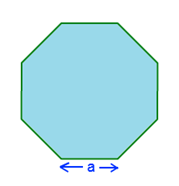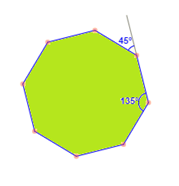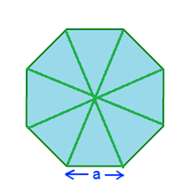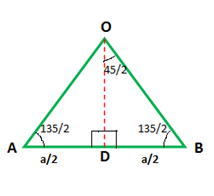# Area Of An Octagon Formula

You know that polygons are 2D closed figures which are made up of 3 or more line segments. Triangle is the smallest polygon with 3 sides. Similarly, the polygon which has 8 sides is called an octagon. If the 8 sides of the octagon are equal in length and the angles between these sides are equal, the octagon is called a regular octagon. In a regular octagon, the measure of each of the interior angle is 135 degrees. The exterior angles measure 45 degrees each.

In this section, you will learn how to calculate the area of an octagon. Also, how to derive a formula for the same and solve some examples so as to give you a clear understanding of the concept.

Now, the formula for computing the area of a regular octagon can be given as:Area of an Octagon =

$$\begin{array}{l}2a^{2}(1+\sqrt{2})\end{array}$$

Where ‘a’ is the length of any one side of the octagon.

Properties of a Regular Octagon:

Before we go ahead and derive the area of an octagon formula, let us go through some of the basic properties of a regular octagon.

• It has 8 sides and 8 interior angles.
• All the sides are equal in length and all the angles are equal in measurement.
• There are a total of 20 diagonals in a regular octagon.
• Each interior angle measures 135 degrees. Hence, the sum of all interior angles is (135 x 8) = 1080 degrees.
• Each exterior angle measures 45 degrees. Hence, the sum of all exterior angles is (45 x 8) = 360 degrees.Derivation:

For deriving the area formula, let us consider a regular octagon. Each of its sides is of length a units. Mathematically, area of an octagon is defined as the region covered inside the boundary of an octagon.

Let us divide the octagon into 8 small isosceles triangles by joining each vertex to the centre. Now if we compute the area of one of these triangles and multiply it by 8, we will obtain the area of the octagon.

Take any one of the triangles and draw a line from the centre O of the hexagon to the midpoint of the base AB. Name the midpoint as D. You will get a right-angled angle. The base length of the triangle is a since it is nothing but the side of the octagon. Thus, OD forms the height of the triangle OAB.Area of the octagon is calculated by summing up the areas of the 8 triangles, or, 8 x Area of one triangle (OAB).

We know the relation 2 sin²θ = 1- cos 2θ. We can write it as:

$$\begin{array}{l}2 cos^{2} \theta =1+cos\;2\theta\end{array}$$

$$\begin{array}{l}tan^{2}\theta =\frac{1-cos\;2\theta}{1+cos\;2\theta}\end{array}$$

$$\begin{array}{l}tan^{2}(\frac{45}{2})=\frac{1-cos\; 45}{1+cos\; 45}\end{array}$$

$$\begin{array}{l}tan^{2}(\frac{45}{2})=\frac{1-\frac{1}{\sqrt{2}}}{1+\frac{1}{\sqrt{2}}}\end{array}$$

$$\begin{array}{l}tan^{2}(\frac{45}{2})=\frac{\sqrt{2}-1}{\sqrt{2}+1}=\frac{(\sqrt{2}-1)^{2}}{1}\end{array}$$

$$\begin{array}{l}tan(\frac{45}{2})=\sqrt{2}-1\end{array}$$

Since ∠AOD = ∠BOD = 45/2, we can say

$$\begin{array}{l}tan(\frac{45}{2})=\frac{AD}{OD}\end{array}$$

Hence,

$$\begin{array}{l}\frac{AD}{OD}=\sqrt{2}-1\end{array}$$

$$\begin{array}{l}OD=\frac{a/2}{\sqrt{2}-1}=\frac{a}{2}(1+\sqrt{2})\end{array}$$

Area of ∆ OAB =

$$\begin{array}{l}\frac{1}{2}\times AB\times OD\end{array}$$

=
$$\begin{array}{l}\frac{1}{2}\times a\times\frac{a}{2}(1+\sqrt{2})\end{array}$$

=

$$\begin{array}{l}\frac{a^{2}}{4}(1+\sqrt{2})\end{array}$$

Area of the octagon = 8 x Area of ∆ OAB

=

$$\begin{array}{l}8\times \frac{a^{2}}{4}(1+\sqrt{2})=2a^{2}(1+\sqrt{2})\end{array}$$

Hence, we can write the formula:

Area of an Octagon =

$$\begin{array}{l}2a^{2}(1+\sqrt{2})\end{array}$$

### Solved Examples

Question 1: Calculate the area of a regular octagon whose side is 35 cm.

Solution: Given,

Side of the octagon = 35 cm

Area of an Octagon =

$$\begin{array}{l}2a^{2}(1+\sqrt{2})\end{array}$$

Area of an Octagon =
$$\begin{array}{l}2\times 35^{2}(1+\sqrt{2})=5914.82cm^{2}\end{array}$$

Question 2: Find the area of a UFC boxing ring where each side is of length 80 cm.

Solution: Given,

Side of the UFC boxing ring = 80 cm

Area of an Octagon =

$$\begin{array}{l}2a^{2}(1+\sqrt{2})\end{array}$$

Area of the UFC boxing ring=

$$\begin{array}{l}2\times 80^{2}(1+\sqrt{2})=30901.93 cm^{2}\end{array}$$

To solve more problems on the topic, download BYJU’S – The Learning App.